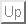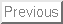Singularities of hyperbolic Gauss maps

Shyuichi IZUMIYA (Hokkaido University)

This is a joint work with D-H. Pei (Northeast Normal University, China)) and T. Sano (Hokkaigakuen University).

In this talk we explain (local) extrinsic differential geometry on hypersurfaces in Hyperbolic space as applications of singularity theory. In 1978, Bleeker and Wilson studied the singularities of the Gauss map of a surface in Euclidean 3-space. In their paper the main theorem asserts that the generic singularities of Gauss maps are folds or cusps. Later that Banchoff et al ,Landis and Platonova have independently studied geometric meanings of cusps of the Gauss map of a surface. Bruce and Romero-Fuster have also independently studied the singularities of the Gauss map and the dual of a hypersurface in Euclidean space. The singularity of the dual of a hypersurface is deeply related to the singularity of the Gauss map of the hypersurface. Their main tools for the study is the height function on a hypersurface. It has been classically known that the singular set of the Gauss map is the parabolic set of the surface and it can be interpreted as the criminant set of the height function. This is the reason why they adopted the height function for the study of Gauss maps. They applied the deformation theory of smooth functions to the height function and derived geometric results on the Gauss maps. We can interpret that these results on Gauss maps describe the contact between hypersurfaces and hyperplanes. It is called the `` flat geometry'' of hypersurfaces in Euclidean space. It also has been known that Gauss maps of hypersurfaces are Lagrangian maps. Moreover, the generic singularities of Gauss maps of hypersurfaces and Lagrangian maps are the same. Singularities of projective Gauss maps are also studied by McCrory et al. There are many other articles concerning on singularities of Gauss maps now.

On the other hand, Epstein (1986) introduced the notion of the hyperbolic Gauss map of a surface in the Poincaré ball model of Hyperbolic space. It is very useful for the study of constant mean curvature surfaces in Hyperbolic space. However, it is very hard to study the singularities of the hyperbolic Gauss map because it is given as an intrinsic expression. Here, we adopt the Hyperboloid in Minkowski space as the model of Hyperbolic space. Under this framework, we can explicitly express the hyperbolic Gauss map (and, indicatrix) of a hypersurface by using the local parametrisation of the hypersurface. In the study of singularities of the hyperbolic Gauss map (indicatrix), we understand that the hyperbolic Guass indicatrix is much easier to proceed the calculation. Corresponding to these notions, we define two hyperbolic invariants. One is the hyperbolic Gauss-Kronecker curvature and another is the hyperbolic mean curvature. We only consider the geometric meaning of the hyperbolic Gauss-Kronecker curvature here. Comparing with the ordinary Gauss-Kronecker curvature, the hyperbolic Gauss-Kronecker curvature is not an intrinsic invariant. It depends on the embedding of the hypersurface into Hyperbolic space. One of our conclusions asserts that the hyperbolic Gauss-Kronecker curvature is a local invariant which describe the contact between hypersurfaces and hyperhoroshperes. This means that we establish the `` horospherical geometry'' of hypersurfaces in Hyperbolic space.